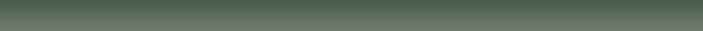Lesson Discounts:Discount Rates are subject to change at the discretion of the Studio.
If this occurs, students will be notified prior to further billing.

The Discounts listed below are for lessons held AT the Studio,
charges may apply for travel and other expenses if lessons are held elsewhere.

***Total Discounts will max out at 50% off regular tuition pricing***

• Paying for lessons in advance, by the month, earns students a 10-15% discount per lesson. (See chart below)

• Any student who takes more than one lesson a week earns a \$5 discount per extra lesson.(No matter the lesson type or lesson length)

• Students who receive good grades can earn FREE LESSONS. Have all A’s and B’s on a report card and earn a FREE Lesson.

• Students who recommend the Studio of Elizabeth Cedroni to a new student receive one FREE LESSON after the new student completes their fourth FULL lesson.(Free lesson Must be used as ONE full-hour lesson)

Rates for Students who pre-pay:

Lesson Type:Lesson Length:Cost:approx. % off:Pre-pay Rate:

Music Lessons     30 minute Lesson\$30/lesson10%\$2745 minute Lesson\$45/lesson12.5%\$4060 minute Lesson\$60/lesson15%\$51

Group Lessons    30 minute Lesson\$40/per session10%\$36
(2-5 students)    45 minute  Lesson\$60/per session12.5%\$5360 minuteLesson\$80/per session15%\$68

Music Studies     30 minute Lesson\$20/lesson10%\$1845 minute Lesson\$30/lesson12.5%\$2660 minute Lesson\$40/lesson15%\$34

Tuition rates:

Intro Lesson (New Students) ...... \$25

Music Lessons (Voice/Piano):
1/2 hour Lesson ...... \$30/lesson
45 minute Lesson...   \$45/lesson
1 hour Lesson ......    \$60/lesson

Group Lessons (2-5 students*):
1/2 hour Lesson ...... \$40/per session
45 minute Lesson...   \$60/per session
1 hour Lesson ......    \$80/per session

Music Studies:
1/2 hour Lesson ……… \$20/lesson
45 minute Lesson...       \$30/lesson
1 hour Lesson ………    \$40/lesson

*The cost of group lessons is shared among the students, the price listed above is the cost
of the lesson as a whole

Payment is expected at the time of lesson or may be paid for in advance. Special privileges are awarded to students who pay for lessons in advance by the month.
For Summer Lesson Rates & New Student Packages